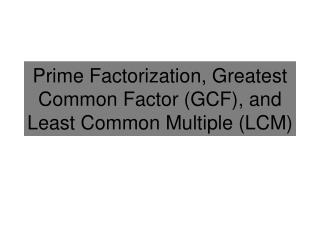DownloadDownload PresentationPrime Factorization, Greatest Common Factor (GCF ), and Least Common Multiple (LCM)

# Prime Factorization, Greatest Common Factor (GCF ), and Least Common Multiple (LCM)

Download Presentation## Prime Factorization, Greatest Common Factor (GCF ), and Least Common Multiple (LCM)

- - - - - - - - - - - - - - - - - - - - - - - - - - - E N D - - - - - - - - - - - - - - - - - - - - - - - - - - -
##### Presentation Transcript

1. Prime Factorization, Greatest Common Factor (GCF), and Least Common Multiple (LCM)

2. Definition of a Prime Number A prime number is a whole number greater than 1 AND can only be divided evenly by 1 and itself. Examples are 2, 3, 5, 7, and 11. Example: Are the following numbers prime? Why or why not? • 31 • 1 • 51 Yes, 31 is greater than 1 AND is only divisible by 1 and 31. No, 1 is not greater than 1. No, 51 is divisible by 1, 3, 17 and 51. Not just 1 and 51.

3. Prime Factorization Prime Factorization is when a number is expressed as a product of prime factors. You can use a factor tree to find the prime factorization of a number. Example: Find the prime factorization of12. 12 2 6 2 3 2

4. Prime Factorization of Algebraic Monomials You can use prime factorization to factor algebraic monomials. A monomial is in factored form when it is expressed as the product of prime numbers and variables and no variable has an exponent greater than 1. Example: Factor 100mn3. 100 mn3 = mnnn 10 10 5 2 5 2

5. Definition of Factors of a Number The factors of a number are all the numbers that can be divided evenly into the number with no remainder. For instance 5 is a factor of 30 because 30 divided by 5 is 6 (a whole number). When finding factors, we typically focus on whole numbers. Example: Find a factor of 48. All the factors of 48 are: 1, 2, 3, 4, 6, 8, 12, 16, 24, 48.

6. Definitions for Common Factors of Numbers Factors that two or more numbers have in common are called common factors of those numbers. Example: Find a common factor of 24 and 30. The largest common factor of two or more numbers is called their Greatest Common Factor(GCF). All the common factors of 24 and 30 are 1, 2, 3, and 6. The GCF of 24 and 30 is 6.

7. Finding a GCF through Reasoning Find the greatest common factor of: 8, 28, 12 Factors of 8: Factors of 28: Factors of 12: Find the largest number that divides all three numbers. The GCF is 4. This process could be long and tedious if the numbers are large. A more efficient method is desirable.

8. Greatest Common Factor (GCF) for Algebraic Expressions The Greatest Common Factor (GCF) for algebraic expressions is the greatest expression that is a factor of the original expressions. Procedure to find the GCF of two or more terms: • Factor each monomial. • The GCF is the product of the common factors.

9. Examples Find the GCF of each set of monomials: • 24, 60, and 72 • 15 and 8 • 15a2b, 9ab2, and 18ab Step One: Factor each Monomial. Step Two: Find the common factors. There is nothing in common, so the GCF is… Step Three: Multiply the factors in common.

10. Definitions for Multiples of Numbers A multiple is the result of multiplyinga number by an integer. A common multiple is a number that is a multiple of two or more numbers. Example: Find common multiples of 3 and 4. The smallest common multiple of two or more numbers is called their least common multiple (GCF). The common multiples of 3 and 4 are 12, 24, 36, 48, etc. The LCM of 3 and 4 is 12.

11. Finding a LCM through Reasoning Find the least common multiple of: 8, 28, 12 Multiples of 8: Multiples of 28: Multiples of 12: Find the smallest number that is a multiple of all three. The LCM is 168. This process could be long and tedious if the numbers are large. A more efficient method is desirable.

12. Least Common Multiple for Algebraic Expressions (LCM) The Least Common Multiple (LCM) for algebraic expressions is the least expression that is a common multiple of the original expressions. Procedure to find the LCM of two or more terms: • Factor each monomial. • Find the greatest number of times each factor appears in each factorization. • The LCM is the product of (2).

13. Examples Find the LCM of each set of monomials: • 18, 30, and 105 • 15a2b and 27b3 Step One: Factor each Monomial. Step Two: Find the greatest number of times each factor appears in each factorization. Step Three: Multiply the result of (2).